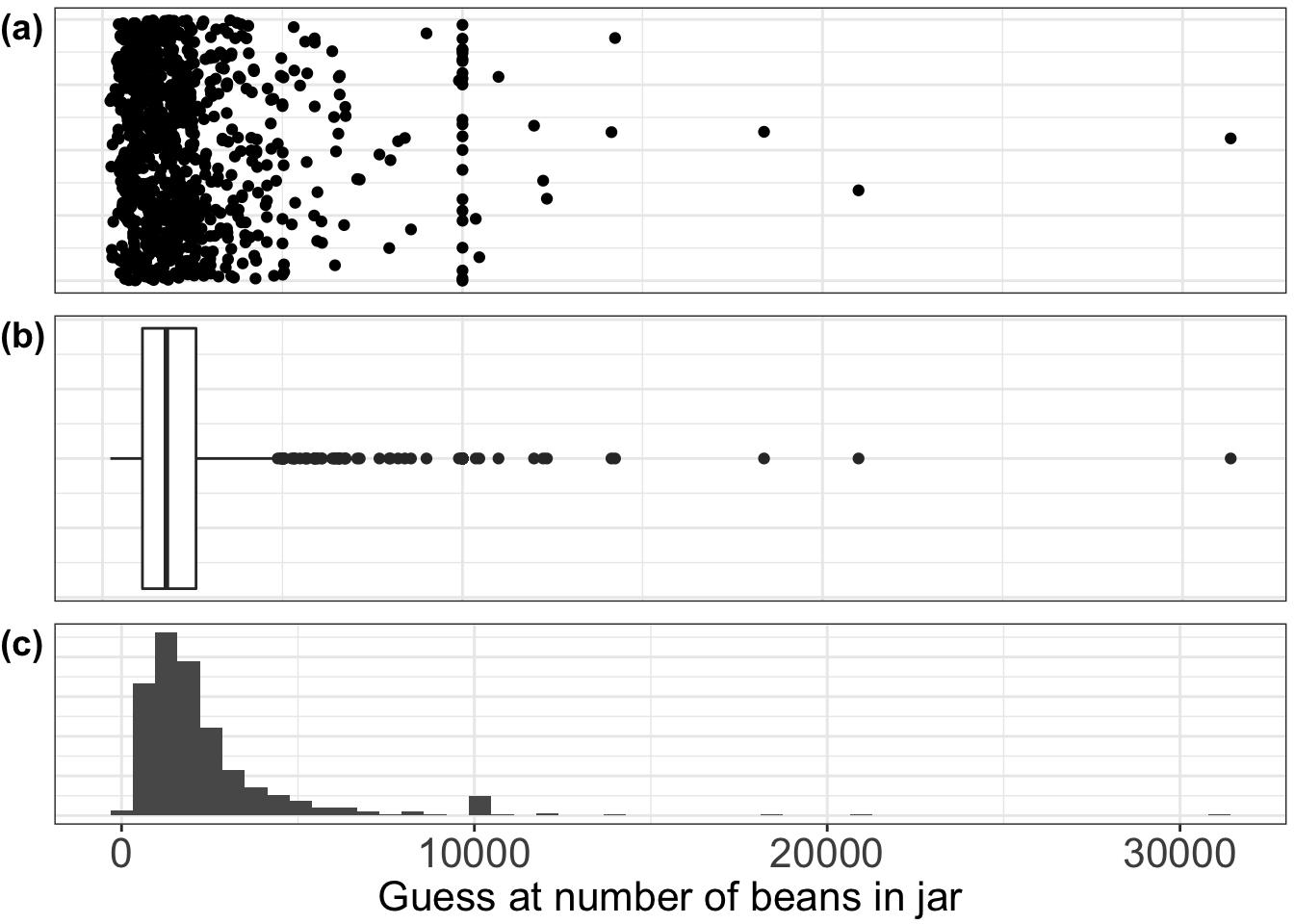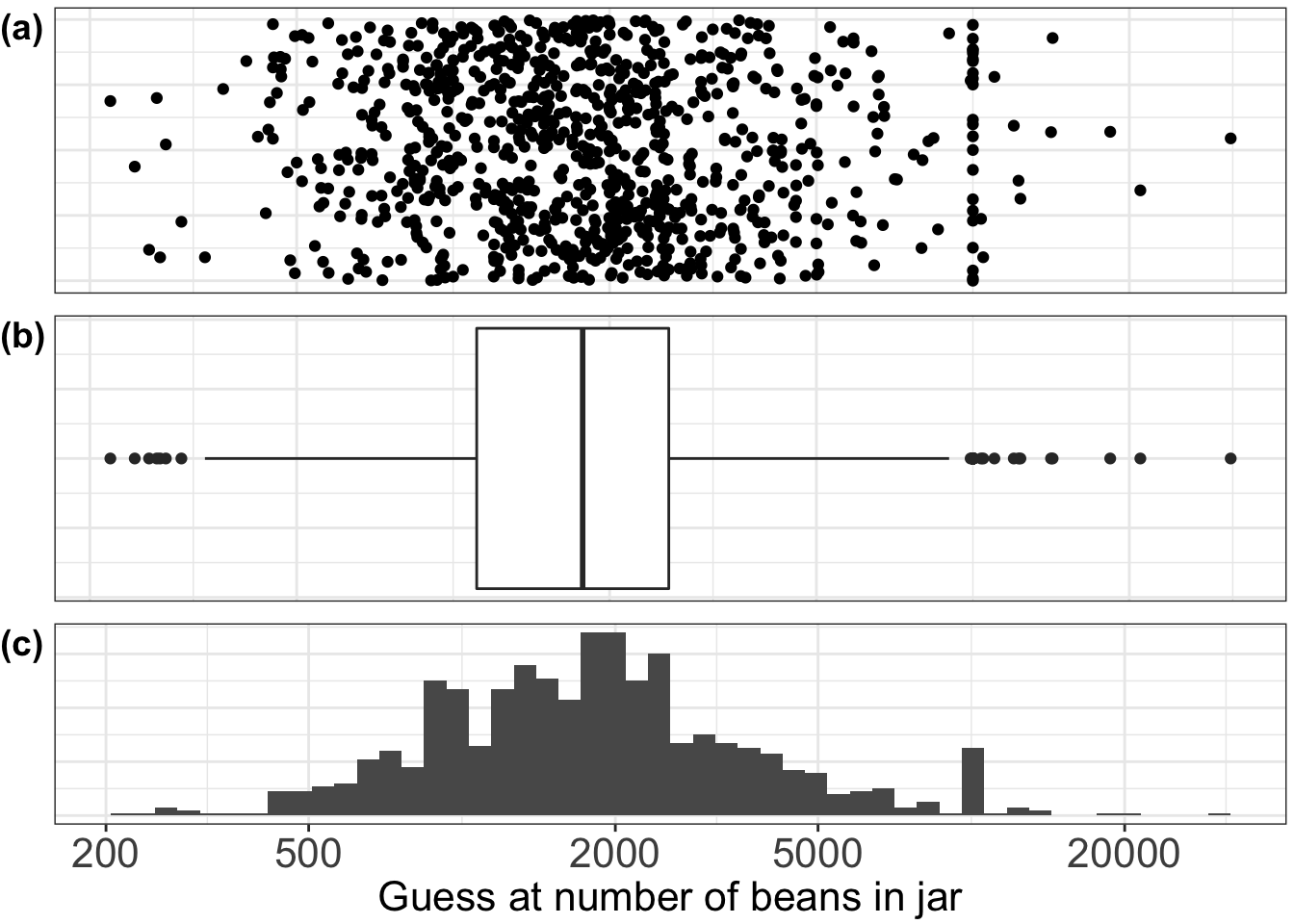Table 2.1: Summary statistics for Jelly bean counts

Data comprise 915 guesses of the numnber of jelly-beans in a jar, and are contained in 02-1-bean-data-full-x.csv.

NGuesses <- nrow(Guesses)
summary(Guesses)
##        V1
##  Min.   :  219
##  1st Qu.: 1110
##  Median : 1775
##  Mean   : 2409
##  3rd Qu.: 2600
##  Max.   :31337
sd(Guesses\$V1) # standard deviation of first column of data-frame
##  2422.171

Figure 2.2: Different displays of Jelly bean counts

library(magrittr)
library("ggplot2")
library("ggpubr")

# load ggplot2 themes into BlankXTheme, BlankYTheme, and BiggerTicks

BlankXTheme <- theme(axis.title.x=element_blank(),
axis.text.x=element_blank(),
axis.ticks.x=element_blank())

BlankYTheme <- theme(axis.title.y=element_blank(),
axis.text.y=element_blank(),
axis.ticks.y=element_blank(),
plot.margin=unit(c(0.1,0.1,0.1,0.65),"cm")) # also give a little space for plot labelling

BiggerTicks <- theme(axis.text.x = element_text(size=16), axis.title.x = element_text(size=16))

AugmentedGuesses <- cbind(Guesses, runif(NGuesses), rep(1, NGuesses))
names(AugmentedGuesses) <- c("Guess", "Aug", "Group") # Aug is jittered plotting position

Strip <- ggplot(AugmentedGuesses, aes(x=Guess, y=Aug)) + geom_point() + labs(y="") + theme_bw() +
BlankXTheme + BlankYTheme # scatter type plot

Box <- ggplot(AugmentedGuesses, aes(x=Group, y=Guess)) + geom_boxplot() + coord_flip() + theme_bw() +
BlankXTheme + BlankYTheme # box and whisker type plot

Hist<- ggplot(AugmentedGuesses, aes(x=Guess)) + geom_histogram(bins=50) + theme_bw()  + BlankYTheme + BiggerTicks + labs(x="Guess at number of beans in jar", hjust=0.0) # histogram plot

p <- ggarrange(Strip, Box, Hist, ncol=1, nrow=3,
labels=c("(a)","(b)","(c)"), hjust=0.0) # arrange in grid with a,b,c labels for the plots,
pFigure 2.2 Different ways of showing the pattern of 915 guesses of the number of jelly beans in the jar. (a) A strip-chart or dot-diagram, with a jitter to prevent points lying on top of each other; (b) a box-and-whisker plot; (c) a histogram

Figure 2.3: Different displays of Jelly bean counts - logarithmic scale

logbreaks <- c(200,500,2000,5000,20000)

Strip <- ggplot(AugmentedGuesses, aes(x=Guess, y=Aug)) + geom_point() +
scale_x_continuous(trans="log10", breaks=logbreaks) +
theme_bw() + BlankXTheme + BlankYTheme # scatter type plot

Box <- ggplot(AugmentedGuesses, aes(x=Group, y=Guess)) + geom_boxplot() +
scale_y_continuous(trans="log10", breaks=logbreaks) +
theme_bw() + BlankXTheme + BlankYTheme + coord_flip() # box and whisker type plot

Hist<- ggplot(AugmentedGuesses, aes(x=Guess)) + geom_histogram(bins=50) +
scale_x_continuous(trans="log10", breaks=logbreaks) +
theme_bw() + BlankYTheme + BiggerTicks + labs(x="Guess at number of beans in jar") # histogram plot

p <- ggarrange(Strip, Box, Hist, ncol=1, nrow=3,
labels=c("(a)","(b)","(c)"), hjust=0.0) # arrange plots in grid with labels
pFigure 2.3 Graphical displays of the jelly-bean guesses plotted on a logarithmic scale. (a) Strip-chart; (b) box-and-whisker plot; (c) histogram all show a fairly symmetric pattern.